Question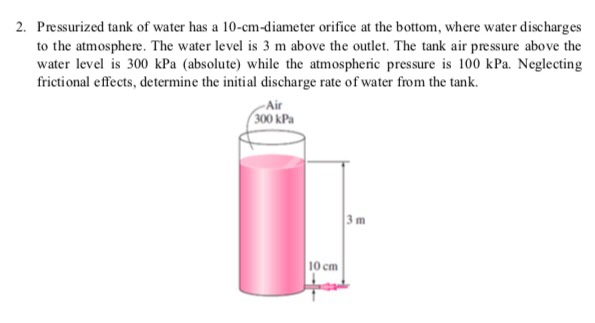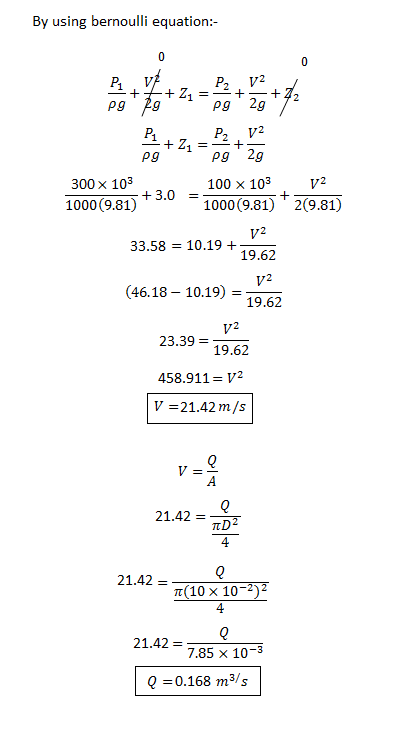#### Earn Coins

Coins can be redeemed for fabulous gifts.

Similar Homework Help Questions
• ### A pressurized tank of water has a 9-cm-diameter orifice at the bottom, where water discharges to...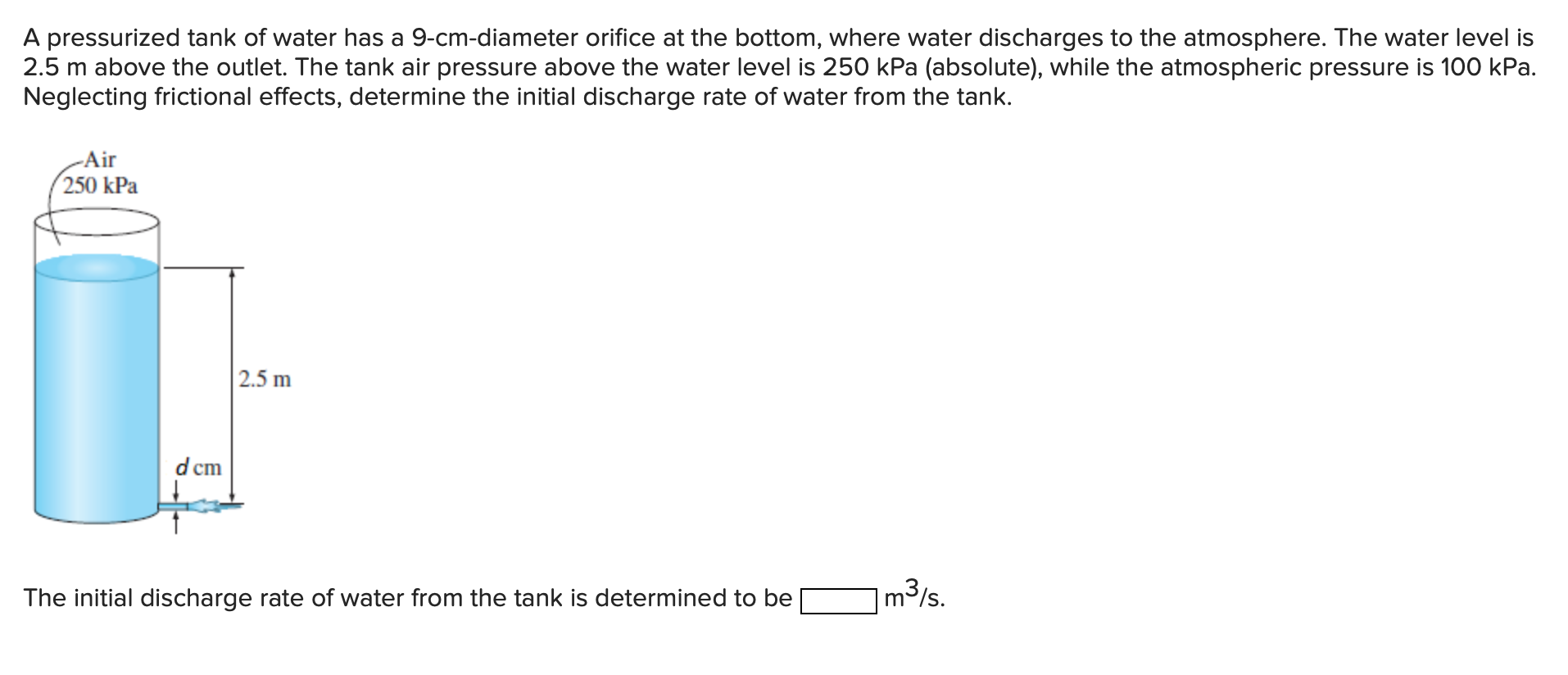A pressurized tank of water has a 9-cm-diameter orifice at the bottom, where water discharges to the atmosphere. The water level is 2.5 m above the outlet. The tank air pressure above the water level is 250 kPa (absolute), while the atmospheric pressure is 100 kPa. Neglecting frictional effects, determine the initial discharge rate of water from the tank. -Air 250 kPa 2.5 m d cm The initial discharge rate of water from the tank is determined to be [...

• ### Air 250 kPa Problem6 A tall tank has an orifice (circular opening) located 2.5 m below...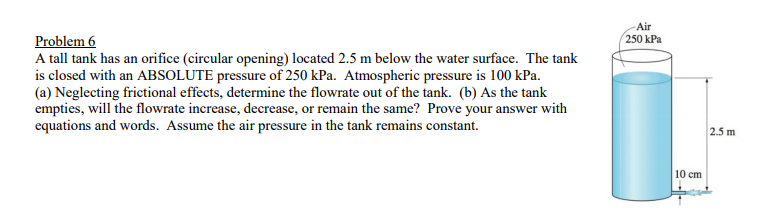Air 250 kPa Problem6 A tall tank has an orifice (circular opening) located 2.5 m below the water surface. The tank is closed with an ABSOLUTE pressure of 250 kPa. Atmospheric pressure is 100 kPa. (a) Neglecting frictional effects, determine the flowrate out of the tank. (b) As the tank empties, will the flowrate increase, decrease, or remain the same? Prove your answer with equations and words. Assume the air pressure in the tank remains constant. 2.5 m 10 cm

• ### Question 8 At the bottom of a pressurized petroleum tank there is a 10 cm diameter...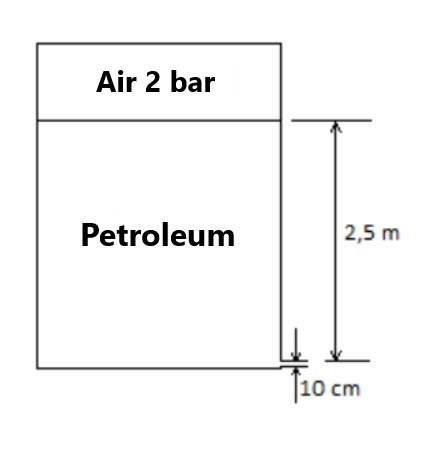Question 8 At the bottom of a pressurized petroleum tank there is a 10 cm diameter hole through which petroleum is poured into the atmosphere. Water level is 2.5m higher than the hole. (Friction losses will be neglected.) 1)Calculate the initial flow of water discharged from the tank. 2)What is the height of the petroleum after 10 seconds? Density of petroleum = 850 kg/m3 Base diameter = 70 cm Atmospheric pressure = 101325 Pa Air pressure on the water surface...

• ### A 3-m-diameter tank is initially filled with water 2 m above the center of a sharp-edged 10-cm-diameter orifice. The tank water surface is open to the atmosphere, and the orifice drains to the at...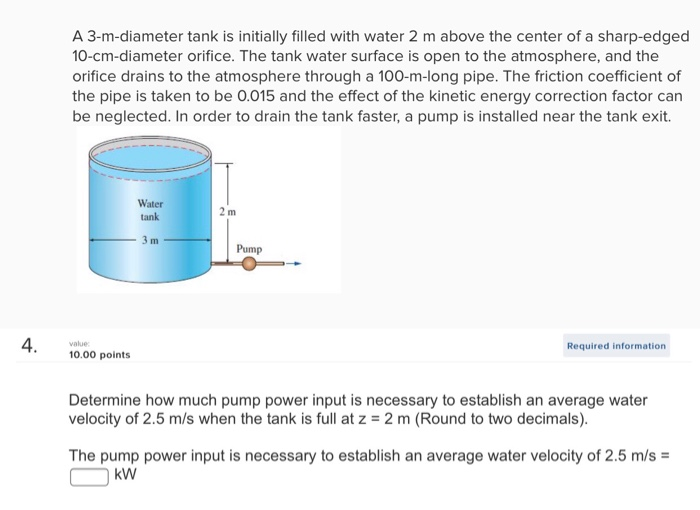A 3-m-diameter tank is initially filled with water 2 m above the center of a sharp-edged 10-cm-diameter orifice. The tank water surface is open to the atmosphere, and the orifice drains to the atmosphere through a 100-m-long pipe. The friction coefficient of the pipe is taken to be 0.015 and the effect of the kinetic energy correction factor can be neglected. In order to drain the tank faster, a pump is installed near the tank exit. Water tank Pump Required...

• ### A 3-m-diameter tank is initially filled with water 2 m above the center of a sharp-edged 10-cm-diameter orifice. The tank water surface is open to the atmosphere, and the orifice drains t...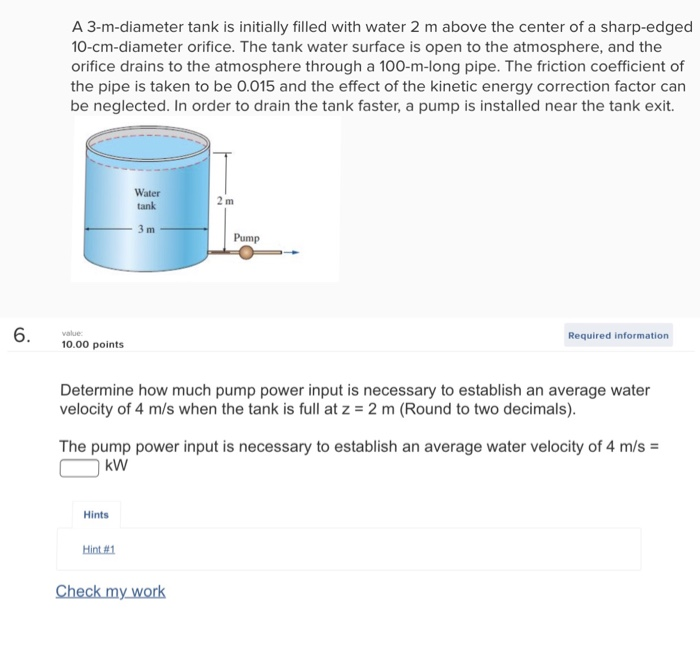A 3-m-diameter tank is initially filled with water 2 m above the center of a sharp-edged 10-cm-diameter orifice. The tank water surface is open to the atmosphere, and the orifice drains to the atmosphere through a 100-m-long pipe. The friction coefficient of the pipe is taken to be 0.015 and the effect of the kinetic energy correction factor can be neglected. In order to drain the tank faster, a pump is installed near the tank exit. Water tank Pump 6...

• ### 7.24 A pump is used to pressurize air in a tank to 300 kPa abs. e tank has a diameter of 2 m and a height of 4 m. Th...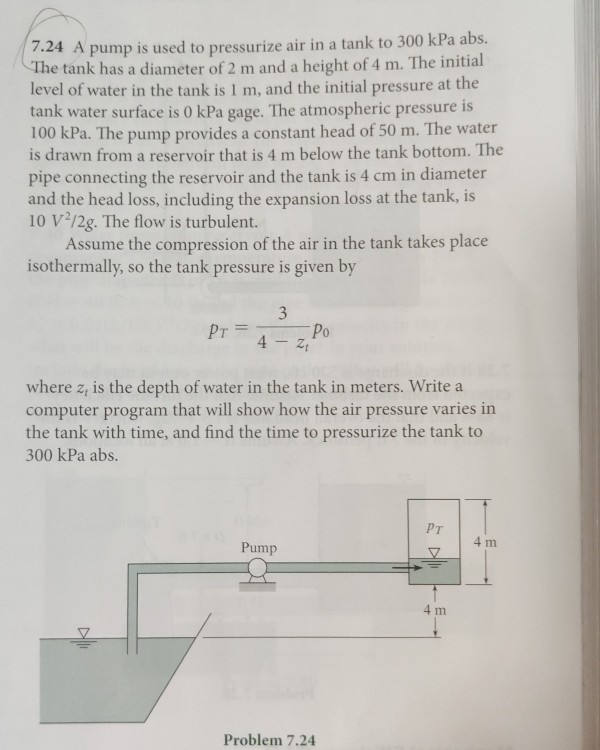7.24 A pump is used to pressurize air in a tank to 300 kPa abs. e tank has a diameter of 2 m and a height of 4 m. The initial level of water in the tank is 1 m, and the initial pressure at the tank water surface is 0 kPa gage. The atmospheric pressure is 100 kPa. The pump provides a constant head of 50 m. The water is drawn from a reservoir that is 4 m below...

• ### Water is siphoned from a large tank and discharges into the atmosphere through a 2-in.-diameter tube...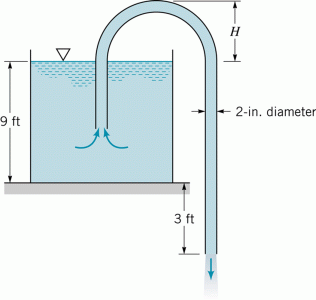Water is siphoned from a large tank and discharges into the atmosphere through a 2-in.-diameter tube as shown in the figure below. The end of the tube is 3 ft below the tank bottom, and viscous effects are negligible. Determine the volume flowrate from the tank. Determine the maximum height, H, over which the water can be siphoned without cavitation occurring. Atmospheric pressure is 14.7 psia, and the water vapor pressure is 0.26 psia

• ### You are assigned the design of a cylindrical, pressurized water tank for a future colony on...

You are assigned the design of a cylindrical, pressurized water tank for a future colony on Mars, where the acceleration due to gravity is 3.71 m/s2. The pressure at the surface of the water will be 150 kPa , and the depth of the water will be 14.1 m . The pressure of the air outside the tank, which is elevated above the ground, will be 91.0 kPa . Find the net downward force on the tank's flat bottom, of...

• ### Question 2 Water is draining from a pressurized tank as shown in the figure. The exit...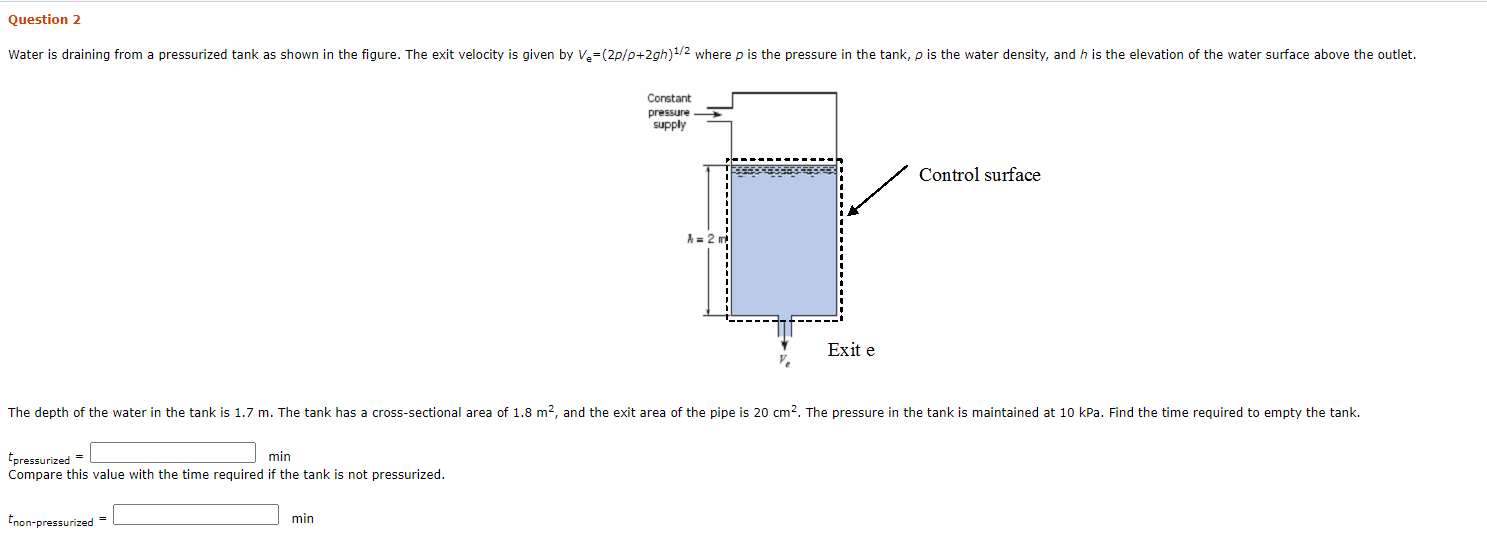Question 2 Water is draining from a pressurized tank as shown in the figure. The exit velocity is given by Ve=(2p/p+2gh)1/2 where p is the pressure in the tank, p is the water density, and h is the elevation of the water surface above the outlet. Constant pressure supply Control surface Exit e The depth of the water in the tank is 1.7 m. The tank has a cross-sectional area of 1.8 m2, and the exit area of the pipe...

• ### The figure shows a cylindrical tank of 80 em in diameter which is fully filled with water. In order to increase the flow from the tank to the exit pipe on the left, an additional pressure is appl...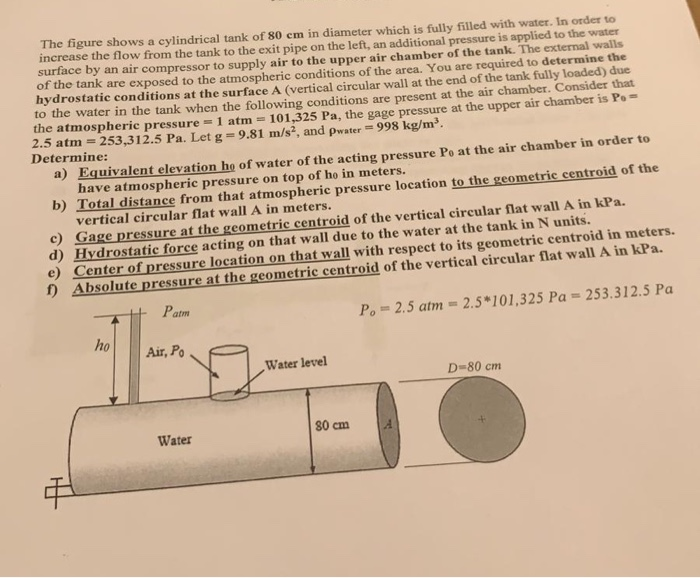The figure shows a cylindrical tank of 80 em in diameter which is fully filled with water. In order to increase the flow from the tank to the exit pipe on the left, an additional pressure is applied to the water surface by an air compressor to supply air to the upper air chamber of the tank. The external walls of the tank are exposed to the atmospheric conditions of the area. You are required to determine the hydrostatic conditions...